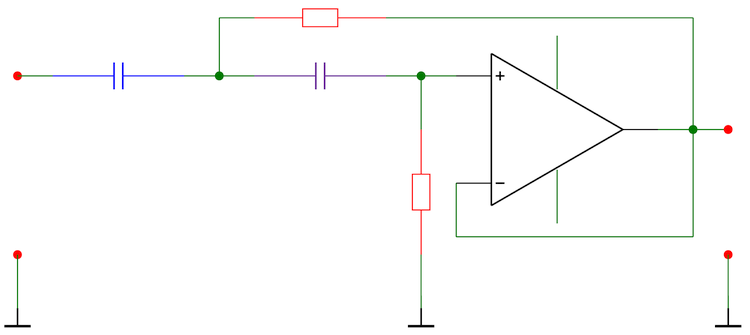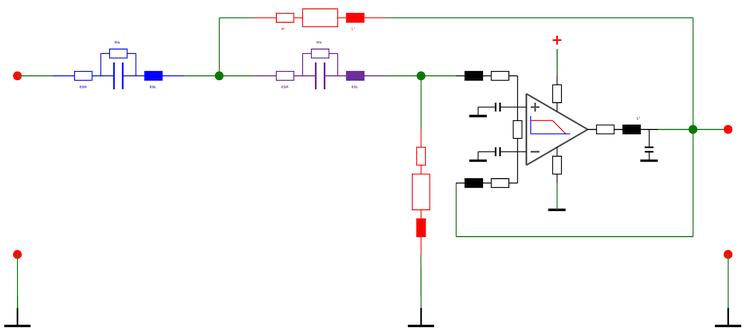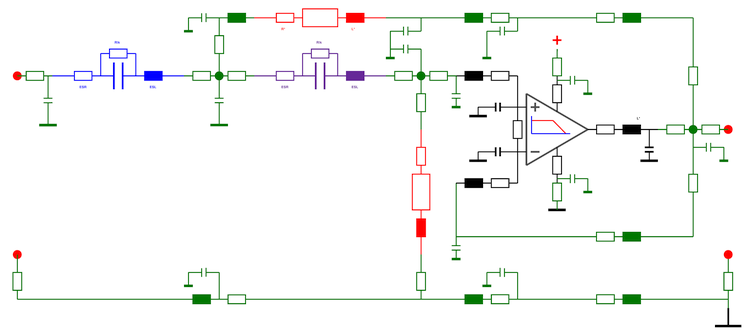www.96kHz.org advanced audio recording Analog Modeling Example Here we deal with analog modelling of an electrical circuit to give an idea of the complexity:   Theoretical view of the analog design:This circuit shows a simple high pass amplifier with an OPA (operational amplifier) using some resistors and condensers and a recoupling loop for stabilization. The Rs and Cs define the edge frequency which easily can be calculated assuming an ideal OPA and ideal components as well. The resulting frequency curve is pretty monotone. The required calculation resources are low because only 5 simple equations are used.   Component View of an analog design:This circuit shows a more complex view on the design, using replacement models of the components for R and C. They take non ideal and high frequency behavior into account while the operational amplifier is still assumed to be ideal and linear except of a little high cut. To do this the correct way the resistors are not only represented by their theoretical value only, but also by additional resistance and inductance because of the physical wire. So are the capacitors containing also bypass conductance. The edge frequency now is not precisely defined anymore and the behavior if the frequency curve is much more tricky. Also the required calculation resources are higher because more then 35 component equations must be solved including a bit OPA modeling.   High frequency view of the analog design:This circuit shows the most appropriate representation of the analog amplifier circuit. It also takes high frequency behavior of the board and the components into account and can comes close to reality for up to 100kHz, even if a linear model with only some high cut is used to evaluate the amplification bandwidth product limit of the OPA. To simulate this, several transmission lines are modeled with a resistance, inductance and capacity. Especially parasitic capacities cause a strong deviation of the real behavior from the simulated simple theory model. The required calculation power is again strongly increased since now nearly 100 equations are used und a smaller time step must be applied during simulation to let the solution converge at all. To get useful results the DSP has to perform about 500 times more arithmetic operations than with the simple theory version. This makes it pretty difficult to simulate analog circuitry precisely enough for real modeling.   © 2003 Jürgen Schuhmacher